# Friction definition | contact force as the cause of friction

Friction / Friction definition: If we either slide or attempt to slide a body over a surface, the motion is resisted by a bonding (cold-weld) between the body and the surface. The resistance is considered to be a force called either the frictional force or simply friction. This force is directed along the surface, opposite to the direction of the intended motion.

## Source of friction

The surface of any object is rough. Even surfaces that feel smooth are covered with microscopic hills and valleys. When two surfaces are touching, the hills and valleys of one surface stick to the hills and valleys of the other surface, as shown in Figure 2.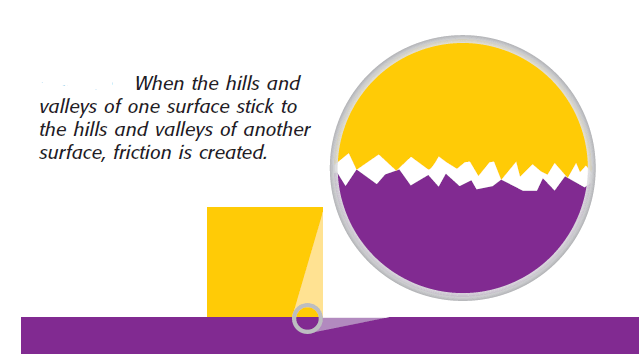fig 2: source of friction – microscopic view of the touching surfaces

This contact causes friction. The magnitude of friction between two surfaces depends on many factors. One factor is the force pushing the two touching surfaces together. Another factor is the materials that make up the surfaces.

### The Effect of weight of the body on Friction

If the force (weight or component of weight) that pushes two touching surfaces together increases, the hills and valleys of the surfaces come into closer contact. This close contact increases the friction between the surfaces. Objects that weigh less exert less downward force than objects that weigh more do, as Figure 3(a) shows.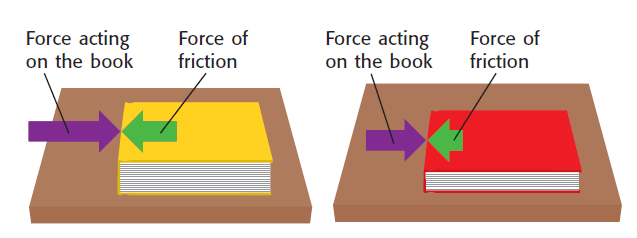figure 3 (a) – The friction between the heavier book and the table is greater than the friction between the lighter book and the table. A harder push is needed to move the heavier book.

But generally, the amount of surface touching another surface does not affect the amount of friction – see figure 3(b) (exception is there though). [ read about race car tires and how it manages friction with larger rubber surface]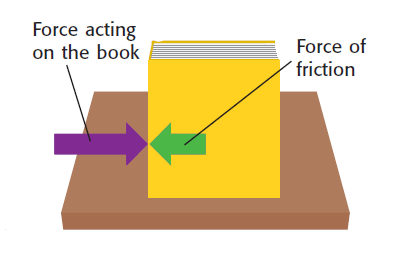figure 3 (b) -Turning a book on its edge does not change the amount of friction between the table and the book.

### The Effect of Material on Friction

The material that makes up a surface affects the magnitude of friction. For example, friction is usually greater between materials that have rough surfaces than it is between materials that have smooth surfaces. A table tennis ball rolling on the carpet slows down and stops because of friction between the ball and the carpet. The amount of friction is large because the carpet has a rough surface. But a table tennis ball rolling on a wood floor rolls for a long time before stopping. The ball keeps rolling because the wood floor has a smooth surface. So, the amount of friction between the ball and the floor is small. Read about friction coefficient.

## How is Friction caused by contact forces between two surfaces?

The following diagram will help us to understand how contact forces between two surfaces give birth to friction.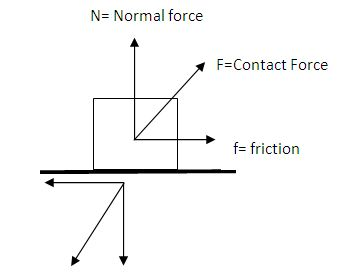figure 4: contact forces and components when we try to move the block towards left from right

Say, there is a block on a surface. (figure 4)

If we intend to move the block towards left from right, then the block will exert a downward push (towards SouthWest in this case) on the surface which makes an angle with the surface.

Now the surface will also apply an equal and opposite force on the block following Newton’s 3rd law of motion.

We have shown the reaction force as F (contact force in the diagram acting upwards towards North-East).

Now if we resolve F along the vertical and horizontal direction, then we find the horizontal component to act opposite to the direction of the intended motion of the block.

So obviously this force is resistive to the intended motion of the block and hence we call it Friction or Frictional force. (labeled in the diagram above)

## How friction resists the Relative motion of the surface below?

Now as the block tends to move here from right to left, the surface below again tends to have a relative motion from left to right. (shown by the red arrow)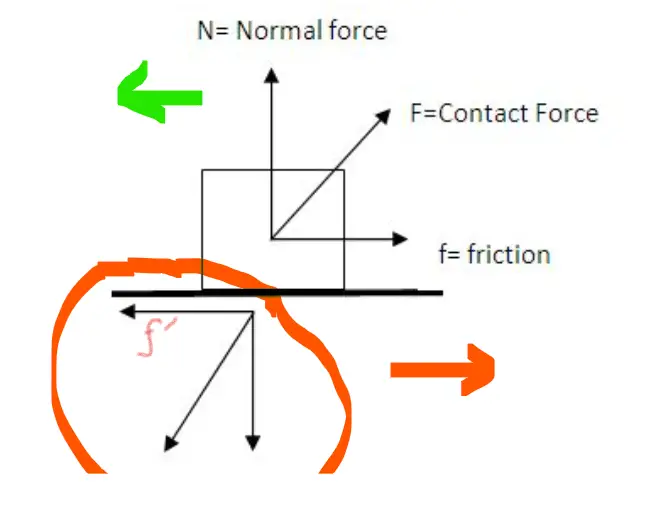figure 5: friction for relative motion of the surface below

So will that relative motion will also face resistive friction?
Yes. How? See here.

Now again we can resolve the downward force exerted on the surface by the block. (circled in red in figure 5)

(This downward force is acting downwards towards SW, we mentioned this one in the previous section as well)

After resolving we see that, the horizontally resolved component is directed towards the left. ( f’ in figure 5)

This is opposite to the tentative relative motion of the surface.

So this is again a frictional force. But this time it acts on the surface below to resist its relative motion.

What we covered here: This topic answers queries like the definition of friction, sources of friction, what is friction, how friction happens between 2 surfaces, etc.

Friction definition | contact force as the cause of frictionScroll to top
error: physicsTeacher.in